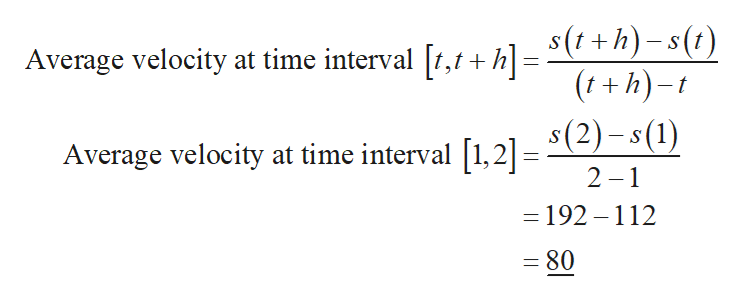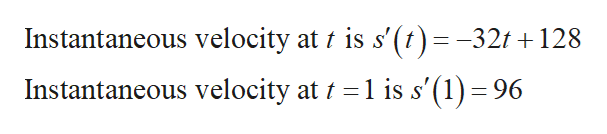# Given the function s(t)= -16t2 + 128t, what is the average velocity at time interval [1,2]? Then make a conjecture about the value of the instantaneous velocity at t=1.

Question
40 views

Given the function s(t)= -16t+ 128t, what is the average velocity at time interval [1,2]? Then make a conjecture about the value of the instantaneous velocity at t=1.

check_circle

Step 1

The given function is,

Step 2

Compute the average velocity at time interval [1,2] as follows.help_outlineImage TranscriptioncloseAverage velocity at time interval [t,t + h]= S(7 +h)-s(1) (t+h)-t _ Average velocity at time interval [1,21= \$(2)-s(1) 2 1 - 192-112 -80 fullscreen
Step 3

Obtain the instantaneous velocity a...help_outlineImage TranscriptioncloseInstantaneous velocity at t is s'(t)=-321 128 Instantaneous velocity at 1 is s'(1) =96 S fullscreen

### Want to see the full answer?

See Solution

#### Want to see this answer and more?

Solutions are written by subject experts who are available 24/7. Questions are typically answered within 1 hour.*

See Solution
*Response times may vary by subject and question.
Tagged in

### Derivative